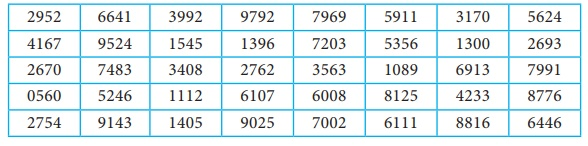Home | | Business Maths 12th Std | Exercise 8.1: Sampling Techniques

# Exercise 8.1: Sampling Techniques

Book back answers and solution for Exercise questions - Maths: Sampling Techniques : Problem Questions with Answer, Solution

Exercise 8.1

1. What is population?

2. What is sample?

3. What is statistic?

4. Define parameter.

5. What is sampling distribution of a statistic?

6. What is standard error?

7. Explain in detail about simple random sampling with a suitable example.

8. Explain the stratified random sampling with a suitable example.

9. Explain in detail about systematic random sampling with example.

10. Explain in detail about sampling error.

11. Explain in detail about non-sampling error.

12. State any two merits of simple random sampling.

13. State any three merits of stratified random sampling.

14. State any two demerits of systematic random sampling.

15. State any two merits for systematic random sampling.

16. Using the following Tippet’s random number tableDraw a sample of 10 three digit numbers which are even numbers.

17. A wholesaler in apples claims that only 4% of the apples supplied by him are defective.

A random sample of 600 apples contained 36 defective apples. Calculate the standard

error concerning of good apples

18. A sample of 1000 students whose mean weight is 119 lbs(pounds) from a school in Tamil Nadu State was taken and their average weight was found to be 120 lbs with a standard deviation of 30 lbs. Calculate standard error of mean.

19. A random sample of 60 observations was drawn from a large population and its standard deviation was found to be 2.5. Calculate the suitable standard error that this sample is taken from a population with standard deviation 3?

20. In a sample of 400 population from a village 230 are found to be eaters of vegetarian items and the rest non-vegetarian items. Compute the standard error assuming that both vegetarian and non-vegetarian foods are equally popular in that village?

17. 0.008

18. 0.9487

19. 0.2739

20. 0.6

21. 0.025

Tags : Problem Questions with Answer, Solution , 12th Business Maths and Statistics : Chapter 8 : Sampling Techniques and Statistical Inference
Study Material, Lecturing Notes, Assignment, Reference, Wiki description explanation, brief detail
12th Business Maths and Statistics : Chapter 8 : Sampling Techniques and Statistical Inference : Exercise 8.1: Sampling Techniques | Problem Questions with Answer, Solution